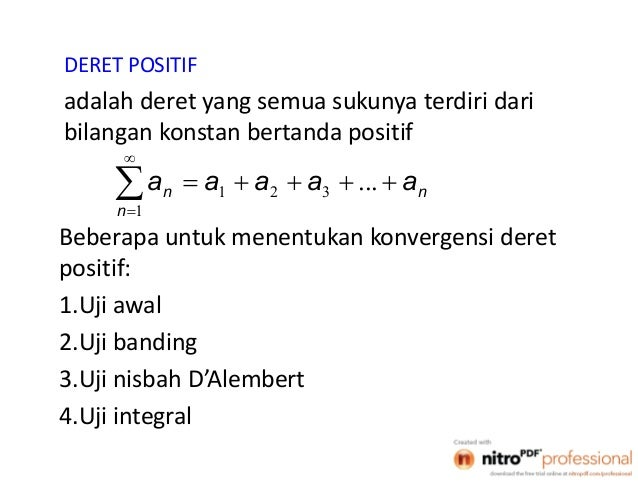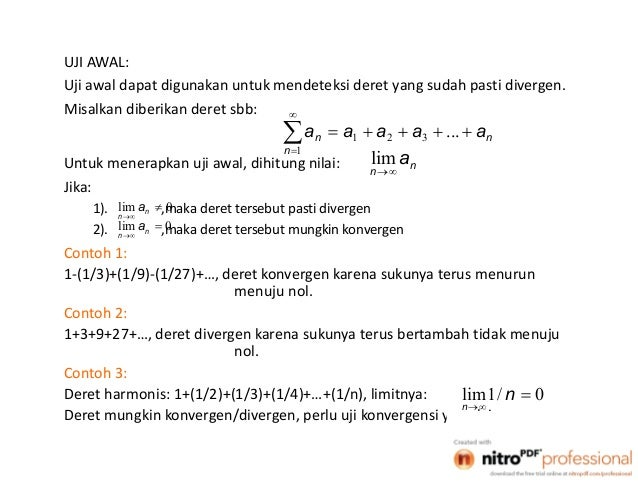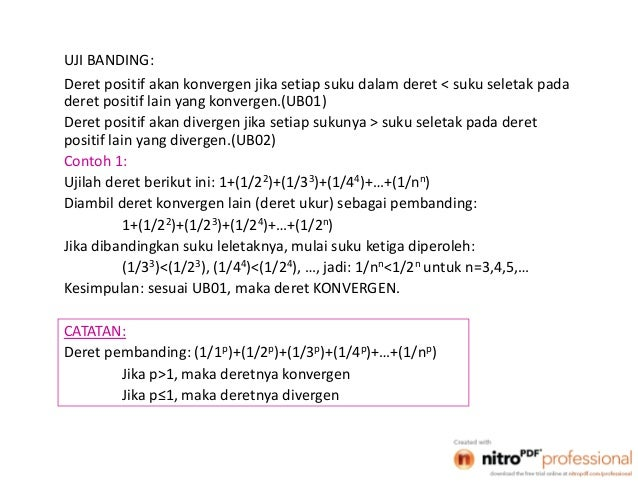Successfully reported this slideshow.Upcoming SlideShare
×

ofUpcoming SlideShare
Rppfiqihviianyar 130722111454-phpapp01(1)
Next

1

Share

# Deret pertemuan ii

Matematika teknik deret

See all

See all

### Deret pertemuan ii

1. 1. 1 DERET POSITIF adalah deret yang semua sukunya terdiri dari bilangan konstan bertanda positif     1 321 ... n nn aaaaa Beberapa untuk menentukan konvergensi deret positif: 1.Uji awal 2.Uji banding 3.Uji nisbah D’Alembert 4.Uji integral
2. 2. 2 UJI AWAL: Uji awal dapat digunakan untuk mendeteksi deret yang sudah pasti divergen. Misalkan diberikan deret sbb:     1 321 ... n nn aaaaa Untuk menerapkan uji awal, dihitung nilai: n n a  lim Jika: 1). ,maka deret tersebut pasti divergen 2). ,maka deret tersebut mungkin konvergen 0lim   n n a 0lim   n n a Contoh 1: 1-(1/3)+(1/9)-(1/27)+…, deret konvergen karena sukunya terus menurun menuju nol. Contoh 2: 1+3+9+27+…, deret divergen karena sukunya terus bertambah tidak menuju nol. Contoh 3: Deret harmonis: 1+(1/2)+(1/3)+(1/4)+…+(1/n), limitnya: Deret mungkin konvergen/divergen, perlu uji konvergensi yang lain. 0/1lim   n n
3. 3. 3 UJI BANDING: Deret positif akan konvergen jika setiap suku dalam deret < suku seletak pada deret positif lain yang konvergen.(UB01) Deret positif akan divergen jika setiap sukunya > suku seletak pada deret positif lain yang divergen.(UB02) Contoh 1: Ujilah deret berikut ini: 1+(1/22)+(1/33)+(1/44)+…+(1/nn) Diambil deret konvergen lain (deret ukur) sebagai pembanding: 1+(1/22)+(1/23)+(1/24)+…+(1/2n) Jika dibandingkan suku leletaknya, mulai suku ketiga diperoleh: (1/33)<(1/23), (1/44)<(1/24), …, jadi: 1/nn<1/2n untuk n=3,4,5,… Kesimpulan: sesuai UB01, maka deret KONVERGEN. CATATAN: Deret pembanding: (1/1p)+(1/2p)+(1/3p)+(1/4p)+…+(1/np) Jika p>1, maka deretnya konvergen Jika p≤1, maka deretnya divergen
4. 4. 4 Contoh 2: Ujilah deret berikut ini: (1/(1.2))+(1/(2.3))+(1/(3.4))+(1/(4.5))+…+(1/(n.(n+1)))+… Jawab: Jika digunakan deret pembanding untuk p=2, maka: (1/12)+(1/22)+(1/32)+(1/42)+… Dari pembandingan suku seletak diperoleh: 1/(1.2)<1/12, 1/(2.3)<1/22, 1/(3.4)<1/32 Kesimpulan: sesuai UB01, maka deret KONVERGEN. …lanjutan
5. 5. 5 UJI NISBAH D’ALEMBERT: Untuk menentukan konvergensi/divergensi suatu deret positif: Caranya sbb (UND): 1.Tentukan suku ke-n dari deret positif tersebut: an 2.Tentukan suku ke-(n+1): an+1 3.Hitung besaran berikut: Jika: <1, maka deret konvergen; >1, maka deret divergen =1, maka deret mungkin divergen, mungkin pula konvergen (uji D’Alembert tidak dapat digunakan)   1n na n n n a a 1 lim    Contoh 1: Ujilah deret berikut ini: (1/1)+(3/2)+(5/22)+(7/23))+…= Jawab: mengacu UND, maka: Sehingga: Kesimpulan: karena <1, maka deret KONVERGEN.     0 2 12 n n n nn n a 2 12   11 2 32    nn n a 2 1 12 2 . 2 32 lim 1      n n n nn 
6. 6. 6 UJI INTEGRAL: Untuk uji konvergensi/divergensi deret positif: dengan uji integral, lebih dulu dihitung: Jika: I bernilai tertentu, maka deret konvergen I=±∞, maka deret divergen Contoh 1: Ujilah deret berikut ini: 1+(1/22)+(1/32)+(1/42))+… Jawab: Bentuk umum suku ke-n: 1+(1/22)+(1/32)+(1/42))+…+(1/n2)= maka: Kesimpulan: karena I=1, maka deret KONVERGEN.   1n na    1 dnaI n   1 2 /1 n n     1 1 2 11/1/1|/1/1 ndnnI
7. 7. 7 LATIHAN: 1. Ujilah kedivergenan deret berikut dengan uji awal: a. 1/3 + 2/5 + 3/7 + … b. 1+ 1/22 + 1/33 +1/44 + … c. 1/2 + 2/3 + 3/4 + … 2. Ujilah kekonvergenan deret berikut dengan uji banding: a. 1/1.2 + 1/2.3 + 1/3.4 + 1/n.(n+1) + … b. 1 + 1/2 + 1/ 3 + … 3. Ujilah kekonvergenan deret berikut dengan uji nisbah d’Alembert: a. ½ + (1/2).(2/22) + (1/3).(3/23) + … b. 1 + 1/2! + 1/3! + … 4. Ujilah kekonvergenan deret berikut dengan uji integral: a. 1 + 1/2 + 1/3 + 1/4 + … b. 1 + 1/4 + 1/9 + 1/27 + …
8. 8. 8 5. Tentukan apakah deret berikut konvergen atau divergen: a. b. c. d. e. …lanjutan    1 2 9 1 n n   2 ln 1 n nn   0 3 2 3 n n   0 !n n n e   2 2/3 1 n n
•#### KronRizki

Feb. 25, 2021

Total views

496

On Slideshare

0

From embeds

0

Number of embeds

6

7

Shares

0